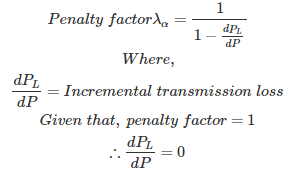# MCQs on Power Systems

##### Page 27 of 67. Go to page 1 2 3 4 5 6 7 8 9 10 11 12 13 14 15 16 17 18 19 20 21 22 23 24 25 26 27 28 29 30 31 32 33 34 35 36 37 38 39 40 41 42 43 44 45 46 47 48 49 50 51 52 53 54 55 56 57 58 59 60 61 62 63 64 65 66 67
01․ In a two plant system, the load is connected to plant number 2. The loss coefficients are
B11,B22,B12 are non zeros
B11 is non zero,B22 = B12 = 0
B11,B12 are non zero ,B22 = 0
B11,B22 are non zero,B12 = 0

Power loss of a transmission line PL = B11×P1² + B22×P2² + 2×B12×P1×P2 Where, B11,B22,B12 and B21 are coefficients P1 and P2 are power delivered by generating stations. When load is connected at plant number 2, the loss coefficients B11 is non zero,B22 = B12 = 0

02․ If the penalty factor of a plant is unity, its incremental transmission loss is
1
-1
0
none of the above03․ Normally Z bus matrix is a
null matrix
full matrix
sparse matrix
unity matrix

Y bus matrix is a sparse matrix, containing more number of zero elements. So that faster calculation is possible. The Y bus matrix is used for the load flow studies. Z bus algorithm or matrix is used for the fault analysis. Inverse of Y bus matrix gives the Z bus matrix, But Z matrix is a full matrix even though Y bus is a sparse matrix. More time is required for inverse of Z bus matrix if the size of the matrix is more than three.

04․ In load flow studies PV bus is treated as PQ bus when
phase angle become high
voltage at the bus become high
reactive power goes beyond limit
any of the above

When a network containing one slack bus, one PV bus and the reactive power value of PV bus is in the out of range for a given value of reactive power limits, so that PV bus is working as PQ bus with the value of Q. For the PQ bus the value of Q is the nearest value from the calculated value of Q in the given range.

05․ As the frequency of the system is increased, the charging MVAR
increases
decreases
remains unaffected
none of the above

Charging MVAR = V*Ic*sinφ Where, V= System voltage Ic = Charging current φ = Phase angle between V and Ic Ic = V/Xc = 2πfC*V Therefore, charging MVAR ∝ f As frequency increases, Charging MVAR also will increase.

06․ The receiving end voltage for a long line under no load condition is
less than the sending end voltage
equal to the sending end voltage
more than the sending end voltage
any of the above

Under no load conditions or light load conditions of a transmission line, receiving end voltage is greater than sending ending voltage will occur in case of medium and transmission lines. This is called ferranti effect. This is due to capacitance effect is dominating compare to inductance of transmission line under no load only. At no load, due to ferranti effect Vr = Vs(1+ω²LC) Increased receiving end voltage ΔVr = Vsω²LC ΔVr = Vs(2πf)²LC*l² = (2πfl)²Vs/V²c Where, Vs = Sending end voltage l = length of transmission line Vc = velocity of signal = 3*10^8 L = inductance of line C = capacitance of line f = frequency

07․ A sort line with R/X ratio 1, the zero regulation is obtained when the power factor of the load is
0.5
unity

Voltage regulation= (Vs - Vr)/Vr = I(R cosφ ± X sinφ)/Vr Where, Vr = Receiving end voltage Vs = Sending end voltage I = Current Condition for zero voltage regulation Tanφ = R/X leading Cosφ = X/Z Z = √(R²+X²) Given that, R/X = 1 Therefore, the power factor of the load is 0.707 leading

08․ Length of short transmission line is up to
80 km
150 km
40 km
any of the above

Classification of transmission lines: Short transmission line = Length(L) < 80 km and product of length(L) and frequency(f) L.f< 4000 Medium transmission line = 80 km ≤ L ≤ 200 Km and 4000 ≤ L.f ≤ 10000 Long transmission line = L > 200 km and L.f >10000

09․ Radio interference occur in telecommunication line due to
electro magnetic induction
electro static induction
both 1 and 2
nether 1 nor 2

If power line and telecommunication lines are running close to each other, the current flowing in the power line produces magnetic flux linkage with the communication line conductor induces an emf in the telecommunication line conductor. This is called electromagnetic induction. Similarly due to earth effect electric field is produced by the charges of the earth induces a voltage in between the conductors of the telecommunication lines. This is called electro static induction. This both electromagnetic induction and electro static induction produces the voltage between the telecommunication line conductors which causes interference to the telecommunication signals which is called radio interference. To reduce this radio interference in the telecommunication line, either power line or both power and telecommunication lines are transposed at regular intervals of length the transmission line.

10․ Which of the following is/are base load plants?
diesel plants
wind plants
nuclear plants
all of the above

Base load plants: A base load power plant is a power station that usually provides a continuous supply of electricity throughout the year with some minimum power generation requirement. Base load power plants will only be turned off during periodic maintenance, upgrading, overhaul or service. Base load power plant has the character of slow demand respond, a mechanism to match generation with the load it supplies. Some examples of base load plants are 1. Thermal plants 2. Hydro plants 3. Nuclear plants 4. Tidal power plants

<<<2526272829>>>